### X.3.6.1 Dimensional point model

We have seen in chapter 3 that an object means a distribution of at least one property on a support domain, an invariant distribution, evaluated against an internal reference system. For making a differentiation between two or more objects with a simultaneous existence, those objects must be distinguished through at least one differential attribute (see the conditions 3.1.a…d from the section 3.1). We have also noticed that a realizable point means a truncated numerical value (which in this paper is named a normal value), and a virtual point is considered as an AAV. Since it is perceived like an elementary object (a distribution element), the realizable point has a finite interval (non determination, uncertainty, error interval) as its support domain, on which its existential attribute is evenly distributed, and the virtual point has a single AAV from the set {R} with its existential attribute, namely a Dirac-type virtual distribution, with a null no determination interval.

Fig. X.3.6.1.1

The figure X.3.6.1.1 shows a graphical plotting of the two cases, the axis X being the representation for the axis of the real numbers {R}. According to this representation, xV corresponds to a singular virtual value (a Dirac-type distribution with an internal reference in AAV xV), while xR1 and xR2, each with a non determination interval, corresponding to two realizable values. We are reminding you that the amount ofis given by the following relation: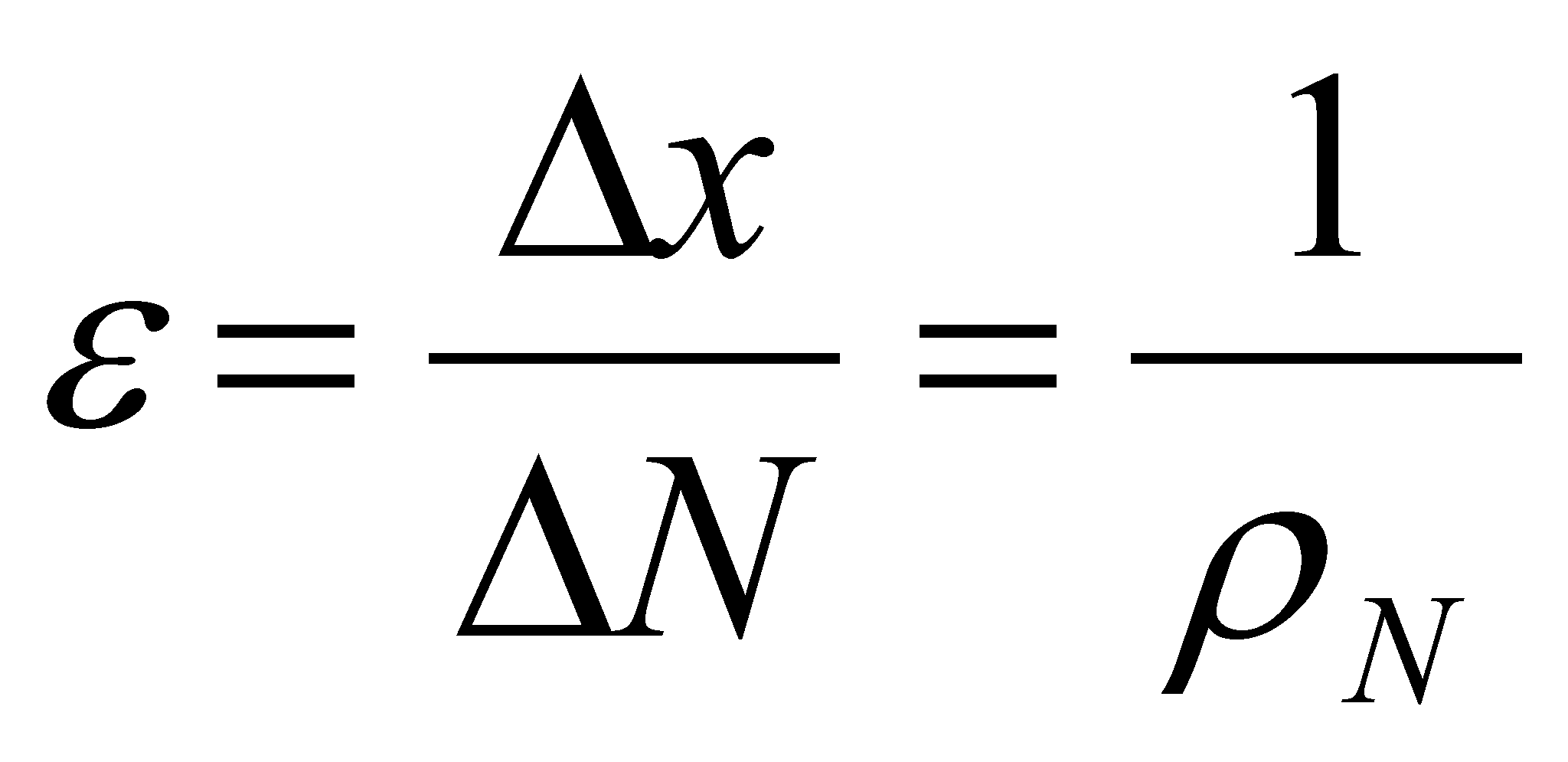(X.3.6.1.1)

that is identical with X.3.1.1.2, where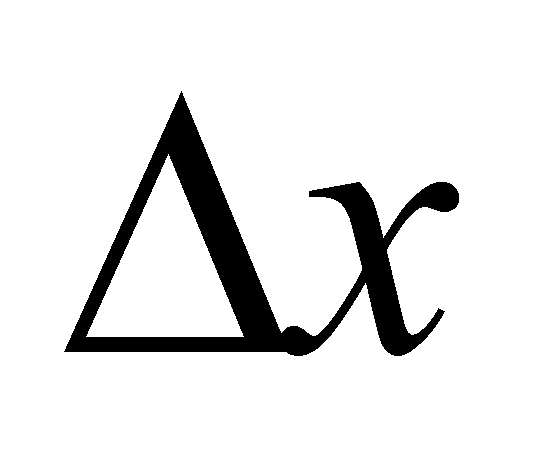is the amount of a finite interval belonging to the axis X and ΔN is the number of singular distinct values from this interval. The amount, which is equivalent to a density (as we saw in section X.3.1.1.) represents the number of the singular values on the axis unit interval, number which is always finite in case of the realizable set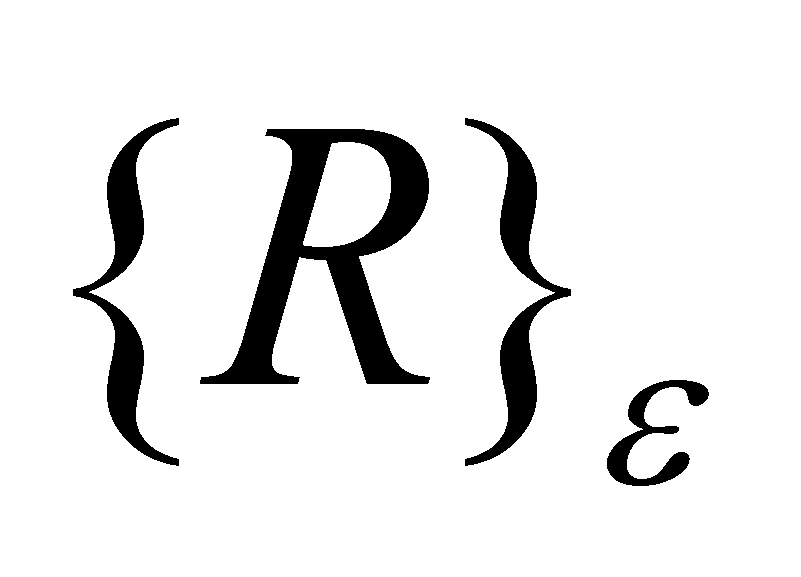.

If we are returning to the values xR1 and xR2 from the figure X.3.6.1.1, they represent, as we already said it, two normal values, even distributions of the existential attribute on the support range, with internal references which are also AAV, but this time, they are realizable by means of a special truncation convention. Because any interval must be defined as an amount, due to its two known boundaries, in case of the singular realizable values, the no determination interval is given either by the difference between two such successive normal values, in this case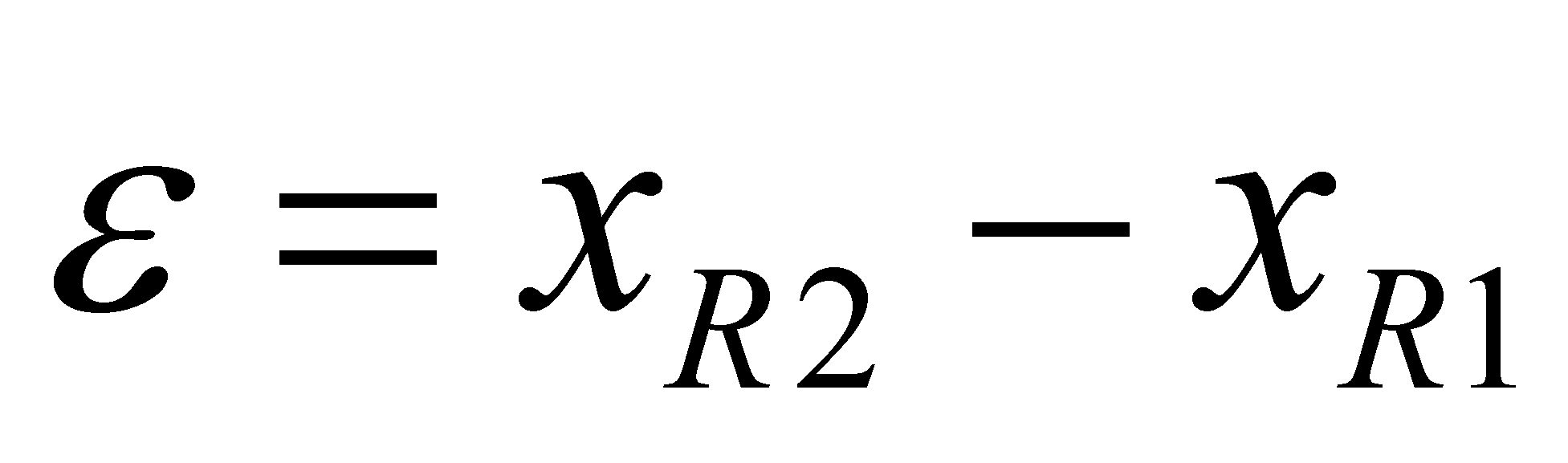, or by a value imposed for the number. According to the above-mentioned issues, xV correspond to a virtual point, and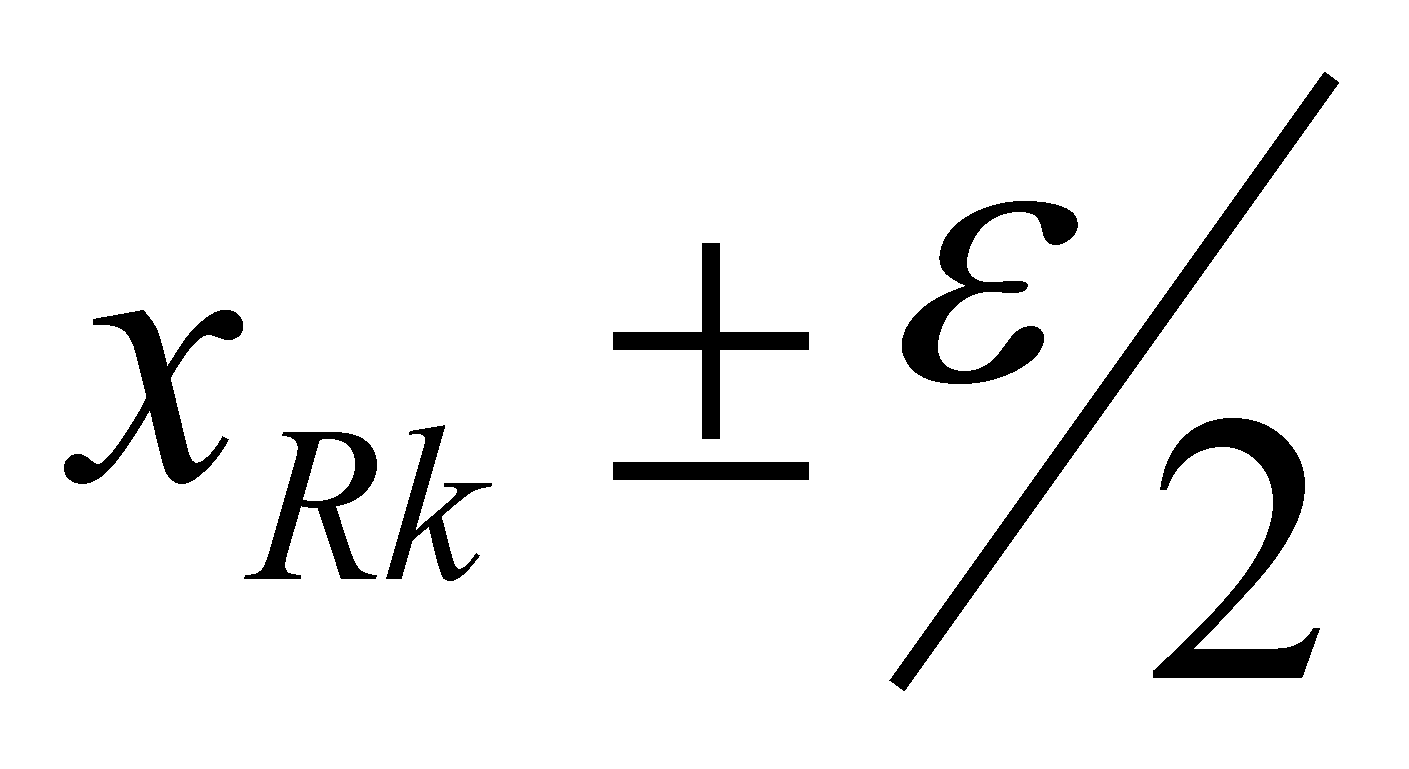to a realizable point with a central internal reference.

Comment X.3.6.1.1: Now, it is time for us to give an explanation to an evident contradiction occurred as a result of introducing the term of realizable AAV. This means that throughout this paper, we shall put the accent on the dichotomy between the abstract realizable and virtual objects and now, we are talking about realizable virtual objects. Well, there is no contradiction, but only a special truncation convention, which allows that the representation to be done only for certain numerical values, of an endless series of digits, by using several special syntax rules. In mathematics, it is well-known the notation rule applied for the decimal numbers with groups of digits which are identically repeated from a certain position after the decimal separator (the so-called decimal periods). For example, 1/3=0,(3), where the group of digits (which in this case is made-up from a single digit) from the brackets is the period. This notation means that the group placed in brackets is endlessly repeated. Other examples: 1/6=0,1(6); 1/11=0,(09); 1/13=0,(076923) and many others. This notation agreement shows a special case: when the decimal period is exclusively made-up from zeros, there are no notations any longer (for instance, 1,(0)=1; 1/4=0,25(0)=0,25 etc.). The numerical values obtained as a result of this latter representation convention shall be named relative accurate values (RAV) because they depend on a truncation convention (of the zeros series). Therefore, if we have an AAV which consists of a finite number of digits followed by an infinite series of zero digits, the renunciation to that infinite zero series makes the representation to be realizable. But, it is only a representation convention, which has to be taken into account - the value from {R} which corresponds to that truncated value contains an infinity of digits (of zeros). For example, this is how the things work in case of the integers; if we would display them accurately as regards the information, as fragments of the set {R} at unit intervals, after their each numerical representation, the decimal separator should follow and it must be followed by an infinite series of zeros. The mutual agreement of representing only the digits which are different from zero placed in front of the decimal separator remains however a truncation form.

Let us return to the point model from the figure X.3.6.1.1 according to the version of the realizable point. In this case, we are also dealing with a known singular value - the internal reference xRk – which is associated to the non determination interval , but this time, the value xRk is a RAV (as we have aforementioned, a special type of AAV which can be represented through the renunciation to an infinite series of zero digits).

The internal reference value of the realizable point may take various positions in the internal domain, but three of them are the most notorious ones: the extreme left, the extreme right and the central positions. The figure X.3.6.1.1 shows the version with the central reference, and in this case, the domainis divided in two sub-domains which are equal on both sides of the reference. The lower boundary of the domain shall be placed at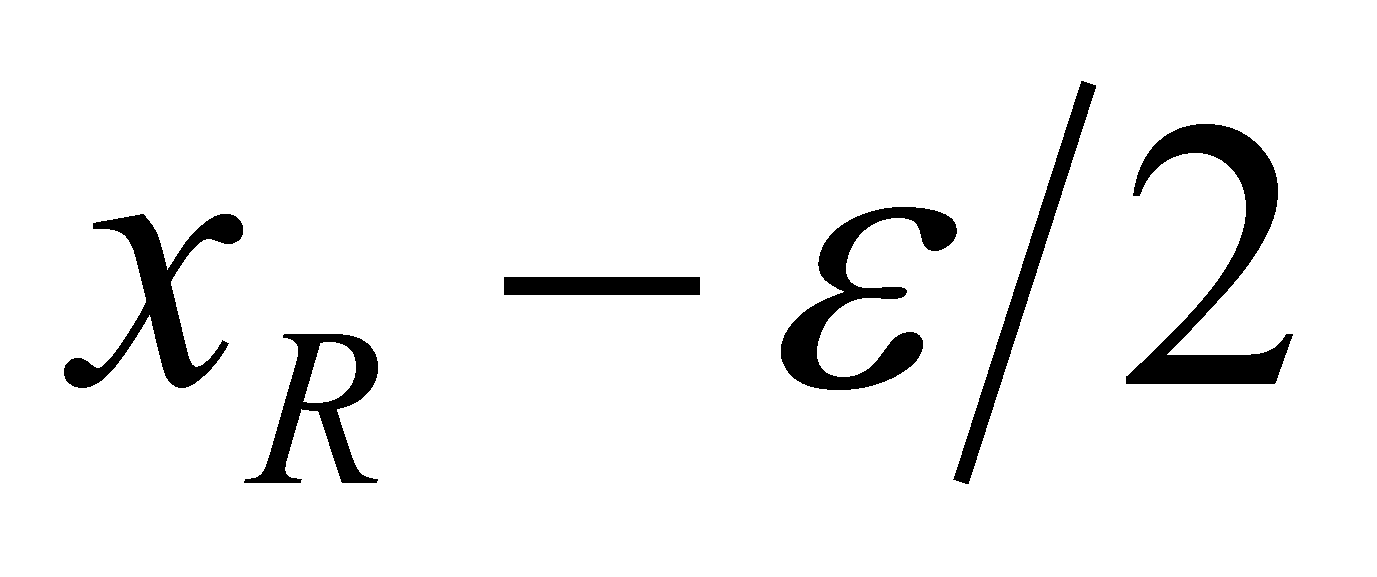, the upper one at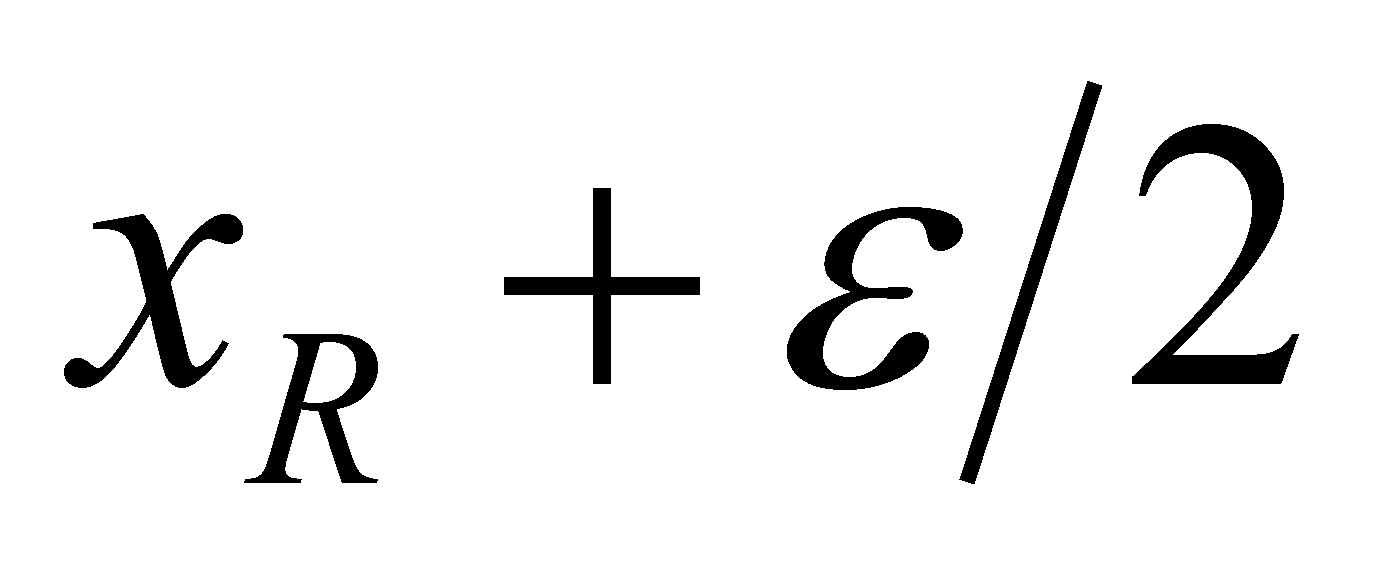, and by using the notation for the open intervals from the mathematics, this domain may be written as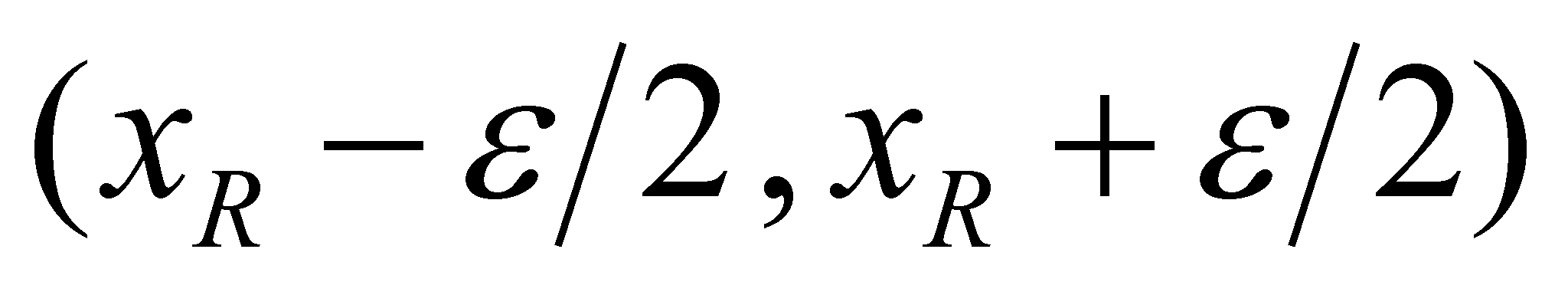. In case of the left or right references, we shall use the notation for semi closed intervals which is very convenient in case of the concatenation of the realizable points. By considering the version of the extreme left reference, the support interval may be written as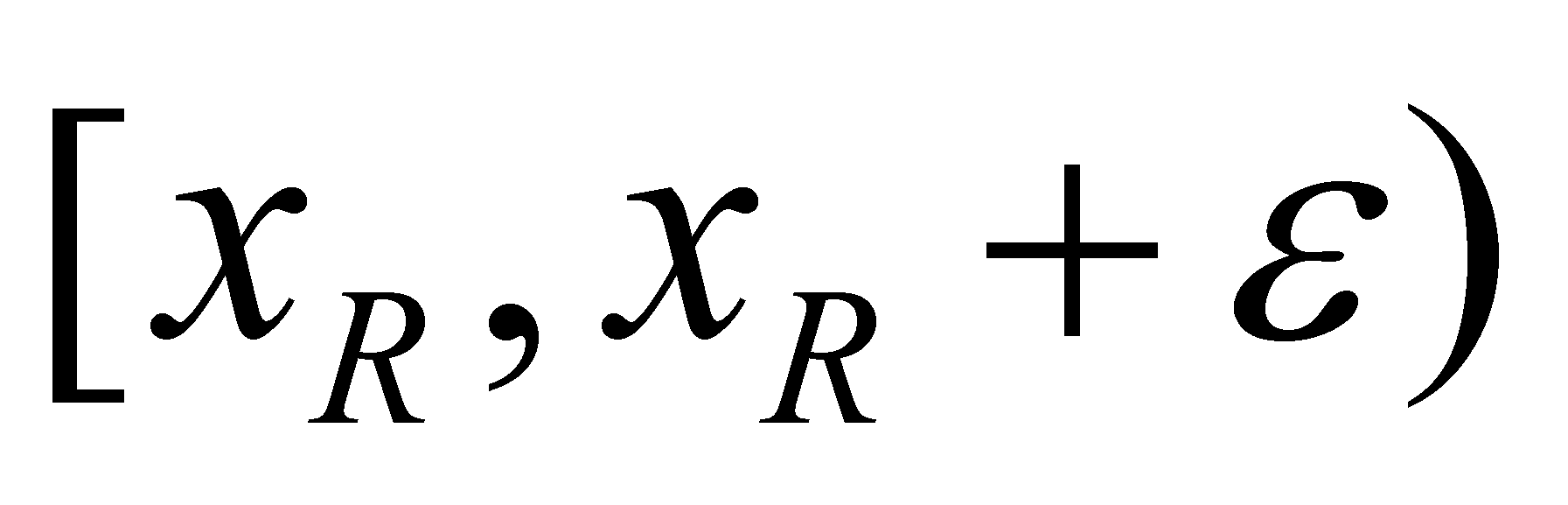, and the extreme right version may be written as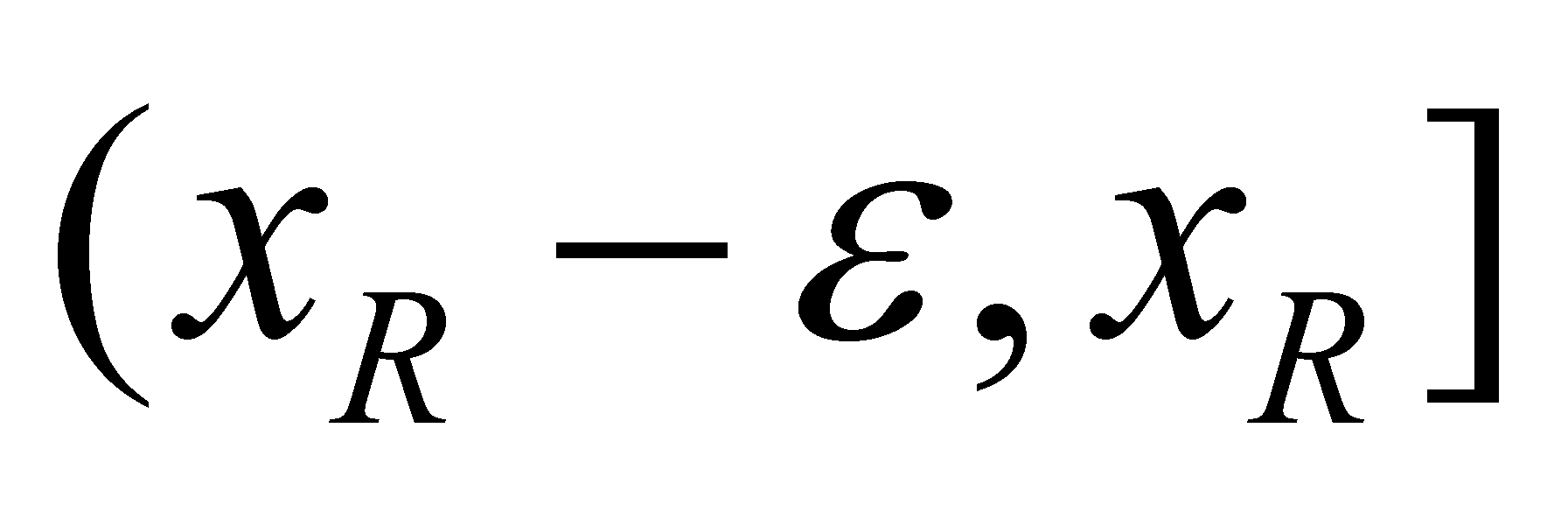.

In case of the realizable point object, as we have already mentioned in chapter 2, the objectual philosophy states a special name, that is the dimensional point (DP). In case of an one-dimensional domain (1D) of a qualitative attribute X, the model of this abstract object may be defined as:

1. Internal domain, truncation interval (no determination, uncertainty)associated to an AAV xR from the set {R}, that is an interval where no other intermediate value can be found and where the existential attribute of DP is evenly distributed;

2. The internal reference of the DP object is that AAV, as a result of the truncation process, which has become a RAV with a finite number of digits;

3. The object’s boundaries are the two values: xu (the upper boundary) and xl (the lower boundary) with values obtained by means of specific relations which depend on the agreement settled upon the internal reference position, which were aforementioned.

By using the literal mathematic syntax for the semi closed intervals of numerical values, the object DP 1D may be displayed according to the version of the extreme left reference, as follows: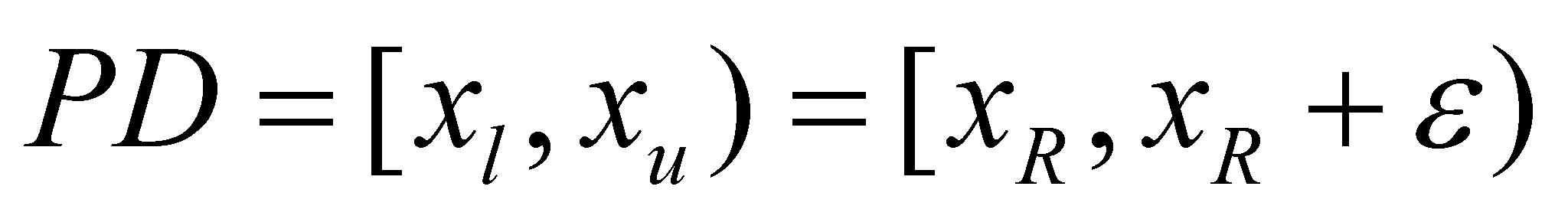(X.3.6.1.2)